# Roman Chijner

•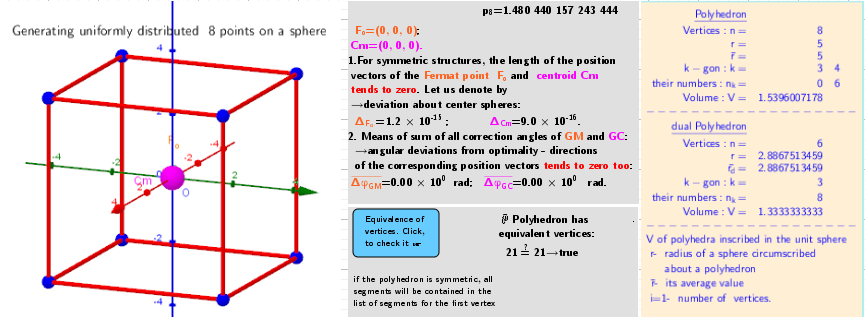### 3. Cube. Polyhedra with extreme distribution of equivalent vertices.

Activity

Roman Chijner

•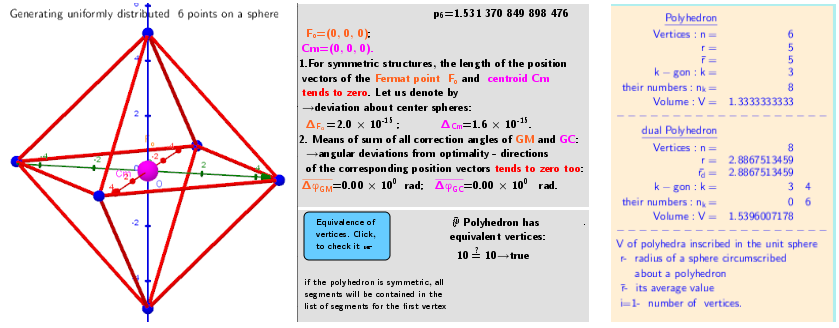### 2. Octahedron. Polyhedra with extreme distribution of equivalent vertices.

Activity

Roman Chijner

•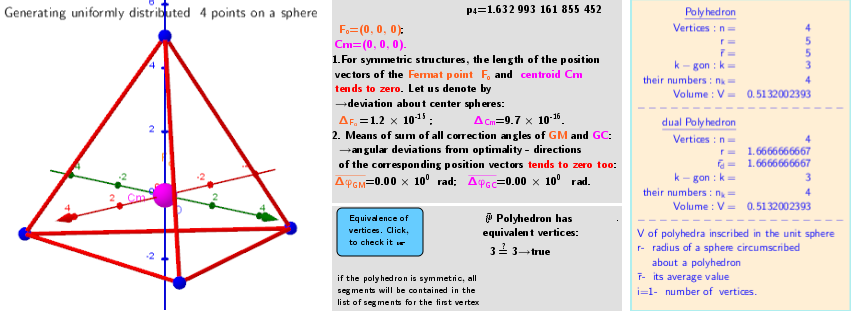### 1. Tetrahedron. Polyhedra with extreme distribution of equivalent vertices.

Activity

Roman Chijner

•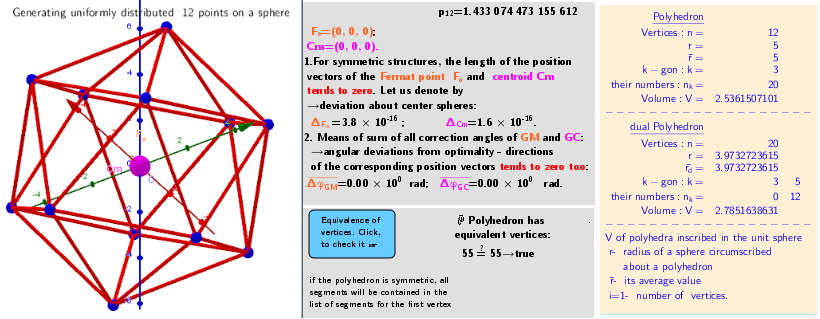### 5. Icosahedron. Polyhedra with extreme distribution of equivalent vertices.

Activity

Roman Chijner

•### Polyhedra whose vertices are equivalent and have an extreme distribution on the same sphere.

Activity

Roman Chijner

•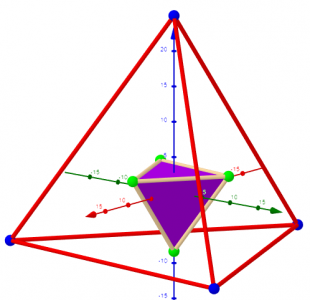### Polyhedra with extreme distribution of equivalent vertices

Book

Roman Chijner

•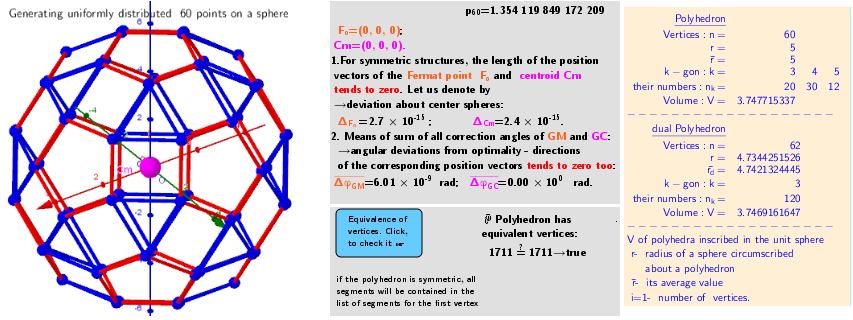### 11. as Rhombicosidodecahedron . Polyhedra with extreme distribution of equivalent vertices.

Activity

Roman Chijner

•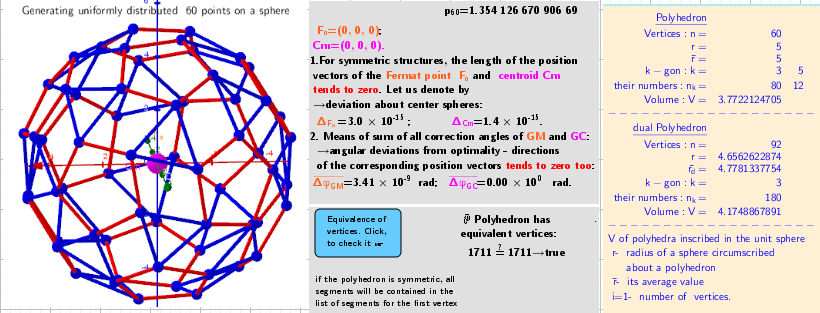### 10. Biscribed Snub Dodecahedron (laevo) . Polyhedra with extreme distribution of equivalent vertices.

Activity

Roman Chijner

•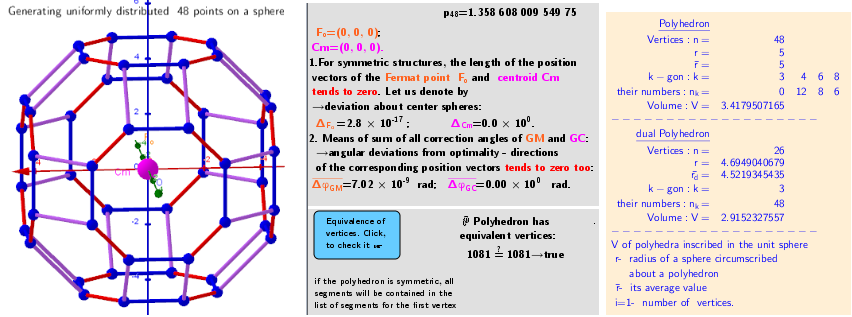### 9. as Truncated Cuboctahedron . Polyhedra with extreme distribution of equivalent vertices.

Activity

Roman Chijner

•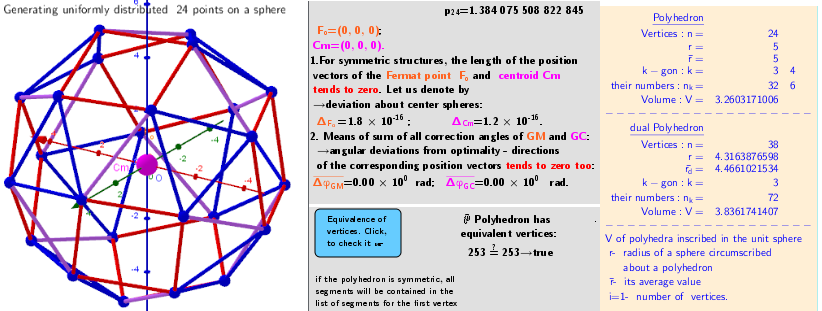### 8. Biscribed Snub Cube(laevo) . Polyhedra with extreme distribution of equivalent vertices.

Activity

Roman Chijner

•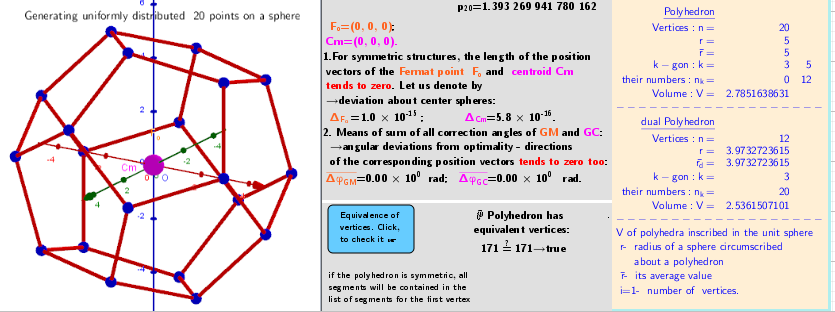### 7. Dodecahedron. Polyhedra with extreme distribution of equivalent vertices.

Activity

Roman Chijner

•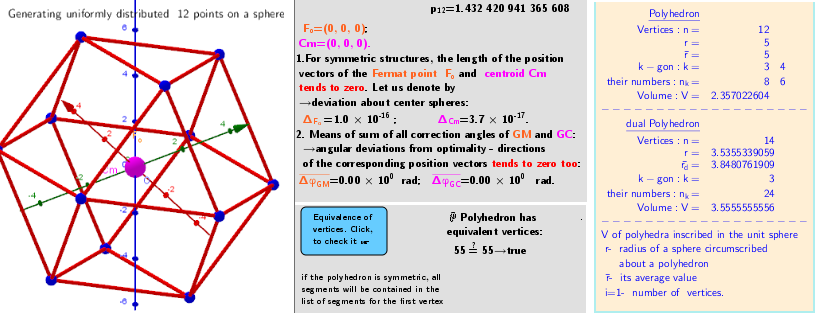### 6. Cuboctahedron. Polyhedra with extreme distribution of equivalent vertices.

Activity

Roman Chijner

•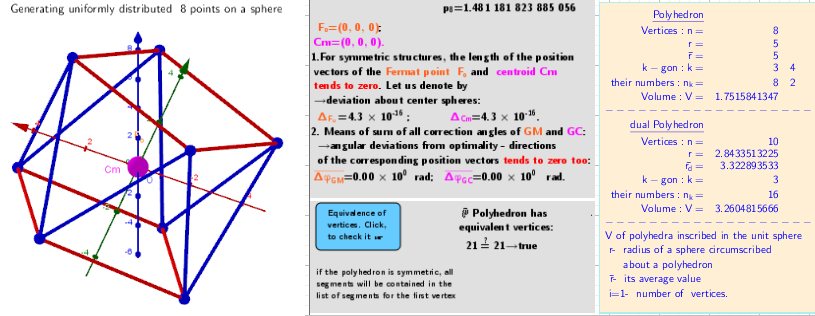### 4. Square antiprism. Polyhedra with extreme distribution of equivalent vertices.

Activity

Roman Chijner

•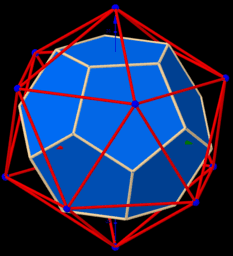### 3 in 1. Constructing, surface triangulation, visualizing polyhedron. New Version.

Activity

Roman Chijner

•### 2. Generating Elements of mesh modeling the surfaces of convex polyhedrons and its dual images

Activity

Roman Chijner

•### inequalities

Book

Roman Chijner

•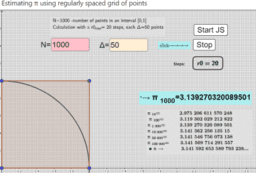### Pi Approximations

Book

Roman Chijner

•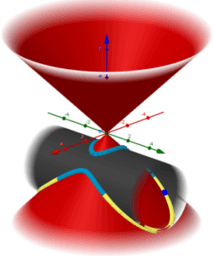### intersection: surface-surface, curve-curve

Book

Roman Chijner

•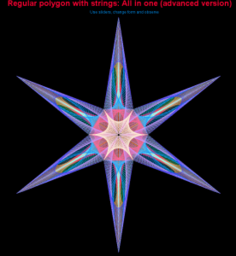### Regular polygon with strings

Book

Roman Chijner

•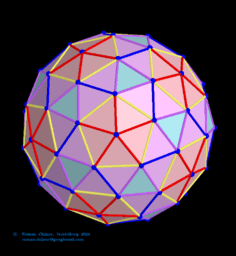### Coloring of edges and faces of a polyhedron(n=72) extreme distribution and its dual image.

Activity

Roman Chijner

•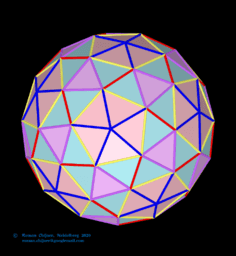### Coloring the edges and faces of a polyhedron(n=72) Biscribed Pentakis Snub Dodecahedron (laevo) and its dual image

Activity

Roman Chijner

•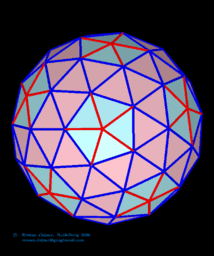### Coloring the edges and faces of a polyhedron(n=72) Pentakis Snub Dodecahedron (laevo) and its dual image.

Activity

Roman Chijner

•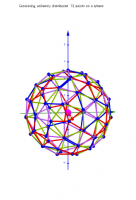### 3d shapes: n=72. Extreme distribution of points on the surface of a sphere

Activity

Roman Chijner

•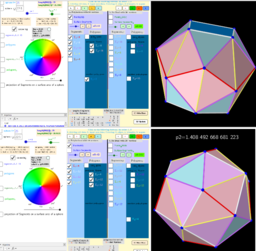### Example of non-uniqueness of the extreme distribution of n=16 particles on the surface of a sphere.

Activity

Roman Chijner